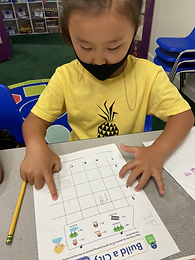Ms. Joan

Target 1​

Lesson Type:

New

Algebra

:

Variable

Determine the unknown number in an addition or subtraction equation relating three whole numbers.

1:

Use understandings of addition and subtraction to solve problems where one addend or subtrahend is given and the sum or difference to solve for the unknown number.

2:

Understand the inverse relationship between addition and subtraction.

Kindergarden

Vocabulary:

Activities:

Students used given information to figure out what number the shapes represents.

Students used the clues to find out what number the shapes and bugs represents.

Students figured out what letters represents by using the given information.Home Exploration

Guiding Questions:Absent Students:

Target 2

:

1:

Understand that in a coordinate, the first number indicates right and left (column) placement and the second up and down (row) placement.

2:

Identify a row and a column.

3:

Use visual tracking to accurately find the intersection point of a row and column.

Kindergarden

Vocabulary:

coordinates, row, column

Activities:

Students made a map of community by drawing pictures on the map according to the coordinates given.Home Exploration

Guiding Questions:Target 3

:

Vocabulary:

Activities:Home Exploration